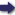(London :  Kegan Paul, Trench, Trübner & Co.,  1910.)

 Tools

## Search this bookPrev Page 38 Next``` 38 ALBERUNTS INDIA. The difference between the two numbers is 43,650,000. If we divide by this number the product of eleven times the universal lunar days, we get as quotient 403,963. Criticisms of This is the number used by the inventor of the this method. "^ . method. It there were not a small remainder beyond the last-mentioned quotient (403,963+a fraction), his method would be perfectly correct. However, there remains a fraction of ^Ws- or s/V, ^^^ t^i^ is the amount which is neglected. If he uses this divisor without the fraction, and divides by it the product of eleven times the partial lunar days, the quotient would be by so much larger as the dividendum has increased. The other details of the calculation do not require comment. Method for Becausc the majority of the Hindus, in reckoning finding the , . • i 77 • /< i • 1 adhimdsa tor their ycars, require the adhimasa, they give the pre- a kalpa, f ercncc to this method, and are particularly painstaking or kaliyuga. ill describing the methods for the computation of the ctdhimdsa, disregarding the methods for the compu¬ tation of the unctrdtra days and the sum of the days (cthargana). One of their methods of finding the ad¬ himdsa for the years of a kalpa or caturyuga or kaliyuga is this:— They write down the years in three different places. They multiply the upper number by lo, the middle by 2481, and the lower by 7739. Then they divide the middle and lower numbers by 9600, and the quotients are days for the middle number and avama for the lower number. The sum of these two quotients is added to the number in the upper place. The sum represents the number of the complete adhimdsa days which have elapsed, and the sum of that which remains in the other two places is the fraction of the current adhimdsa. Dividing the days by 30, they get months. Ya kub Ibn Tarik states this method quite correctly. We shall, as an example, carry out this computation for ourgauge-year. The years of theyfea/^a which have elapsed ```Prev Page 38 Next